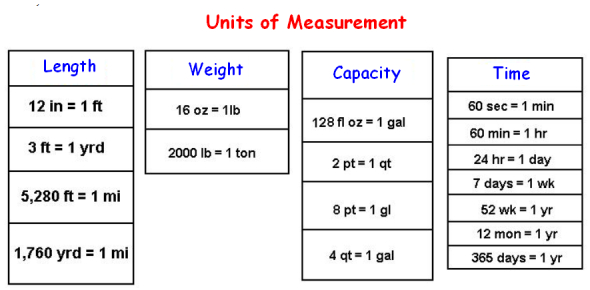# Test Your Units And Measurements Knowledge With The Trivia Quiz

Approved & Edited by ProProfs Editorial Team
At ProProfs Quizzes, our dedicated in-house team of experts takes pride in their work. With a sharp eye for detail, they meticulously review each quiz. This ensures that every quiz, taken by over 100 million users, meets our standards of accuracy, clarity, and engagement.
| Written by Stephen Carpente
S
Stephen Carpente
Community Contributor
Total Contribution - 7 | Total attempts - 18,777
Questions: 10 | Attempts: 7,276SettingsDo you know what are the various units of measurements? Can you convert one measuring unit into the other? If you are confident, why not take this online quiz to test your knowledge and enhance your knowledge along the way. Don't forget to get the certificate of completion at the end of the quiz.
.

• 1.

### Which of the following measurements would NOT be used for measuring the height of someone?

• A.

Centi-metres

• B.

Inches

• C.

Litres

• D.

Feet

• E.

Metres

C. Litres
Explanation
Litre is an SI measurement of VOLUME

Rate this question:

• 2.

### Which of the following is the SI Measurement for Force?

• A.

Joule

• B.

Watt

• C.

Metre

• D.

Second

• E.

Newton

E. Newton
Explanation
Newton is the SI unit for force. It is named after Sir Isaac Newton, a renowned physicist. The Newton is defined as the amount of force required to accelerate a one-kilogram mass by one meter per second squared. It is commonly used to measure the force exerted on an object or the force required to move an object. Other options such as Joule, Watt, Metre, and Second are SI units for energy, power, distance, and time, respectively, and are not directly related to force.

Rate this question:

• 3.

### The SI Unit for time is:

• A.

Second

• B.

Minute

• C.

Hour

• D.

Day

• E.

Year

A. Second
Explanation
The SI unit for time is the second. It is defined as the duration of 9,192,631,770 periods of the radiation corresponding to the transition between the two hyperfine levels of the ground state of the cesium-133 atom. The second is widely used in scientific and everyday measurements of time, and it is the base unit for all other units of time in the International System of Units (SI).

Rate this question:

• 4.

### How many grammes are there in a kilogramme?

• A.

28

• B.

128

• C.

454

• D.

1000

• E.

10000

D. 1000
Explanation
Kilo means 1000

Rate this question:

• 5.

### 238000 Joules would be better expressed as:

• A.

2.38MJ

• B.

238kJ

• C.

23.8GJ

• D.

238mJ

• E.

0.238kJ

B. 238kJ
Explanation
The correct answer is 238kJ because the prefix "k" represents kilo, which is equal to 1000. Therefore, 238kJ is equivalent to 238,000 Joules.

Rate this question:

• 6.

### Convert 1.2m into millimetres.

• A.

12mm

• B.

120mm

• C.

1200mm

• D.

120km

• E.

12km

C. 1200mm
Explanation
To convert meters to millimeters, we need to multiply the given value by 1000 since there are 1000 millimeters in 1 meter. Therefore, 1.2m is equal to 1200mm.

Rate this question:

• 7.

### The new power stations measure their power output in Gigawatts. The power of an electric kettle is measured in kilowatts. How many kilowatts are there is a Gigawatt?

• A.

10

• B.

100

• C.

1000

• D.

1000000

• E.

1000000000

D. 1000000
Explanation
A Gigawatt is equal to 1,000,000 kilowatts.

Rate this question:

• 8.

### Which of the following is a unit of electric current?

• A.

Ohm

• B.

Volt

• C.

Watt

• D.

Amp

• E.

Coulomb

D. Amp
Explanation
The unit of electric current is amp, short for ampere. It is a measurement of the rate at which electric charge flows in a circuit. Ohm is the unit of electrical resistance, volt is the unit of electrical potential difference, watt is the unit of power, and coulomb is the unit of electric charge.

Rate this question:

• 9.

### How many cm are there in 1.59km?

• A.

159

• B.

1590

• C.

15900

• D.

159000

• E.

1590000

D. 159000
Explanation
To convert kilometers to centimeters, we need to multiply the number of kilometers by 100,000. In this case, 1.59 kilometers multiplied by 100,000 equals 159,000 centimeters. Therefore, the correct answer is 159000.

Rate this question:

• 10.

### How many inches are there in 100 yards?

• A.

36

• B.

100

• C.

1200

• D.

3000

• E.

3600Back to top